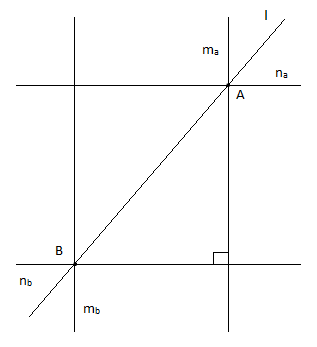# Two distinct points in a plane determine a _____ line.A. Unique.B. Same.C. Parallel.D. Perpendicular.Verified
174.6k+ views
Hint: Recall the postulates / axioms of Euclid's geometry .
Infinitely many lines can pass through any given single point.
Only one line can pass through two distinct points.

The first postulate in the Euclid's Elements, proposes that it is possible to draw a straight line from any point to any point. It means that one, and only one line can pass through some given two points.
There can be infinitely many pairs of parallel or perpendicular lines which can pass through a set of two distinct points.
But two points (distinct) determine a unique line passing through both of them.Notice that the pair of parallel lines ${{m}_{a}}$ and ${{m}_{b}}$ can be rotated by any amount.
Also, the locus of the point of intersection of a pair of perpendicular lines from A and B, is a circle with diameter AB. Therefore, infinitely many pairs of perpendicular lines can pass through A and B.
Only the line $l$ is a unique possibility.
The answer is, therefore, A. Unique.

Note: Infinitely many lines can pass through a single point.
Any two points in space always lie on a unique line. Infinitely many planes can pass through them.
Any three points in space always lie on a unique plane.
Euclid's Postulates:
To draw a straight line from any point to any point.
To produce (extend) a finite straight line continuously in a straight line.
To describe a circle with any center and distance (radius).
That all right angles are equal to one another.
[The parallel postulate]: That, if a straight line falling on two straight lines make the interior angles on the same side less than two right angles, the two straight lines, if produced indefinitely, meet on that side on which the angles are less than two right angles.